The dependence of acceleration due to gravity g on the distance r | FiloClass 12

Physics

Mechanics

Gravitation572

The dependence of acceleration due to gravity on the distance from the centre of the earth, assumed to be a sphere of radius of uniform density is as shown in Fig. below: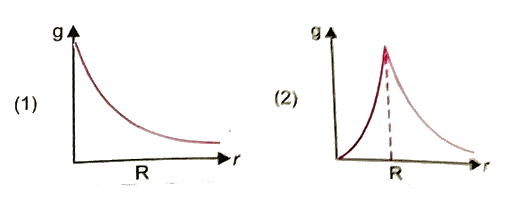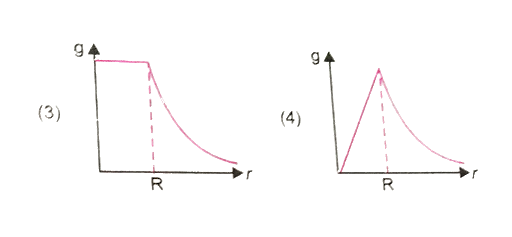The correct figure is

1.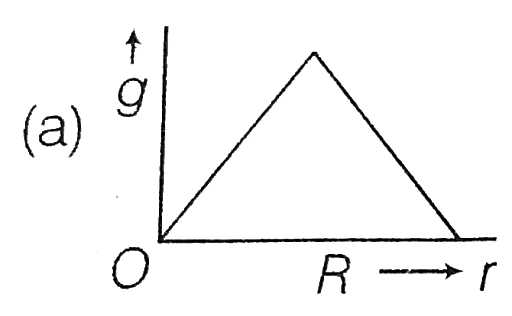2.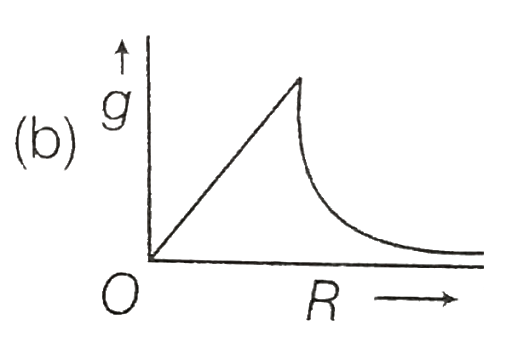3.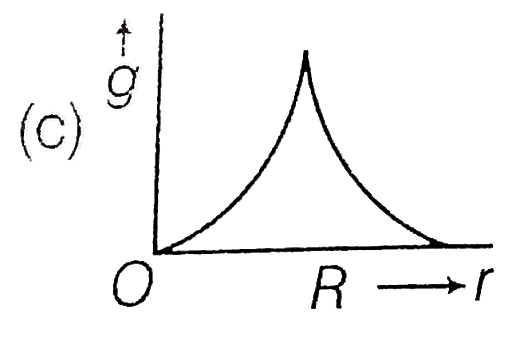4.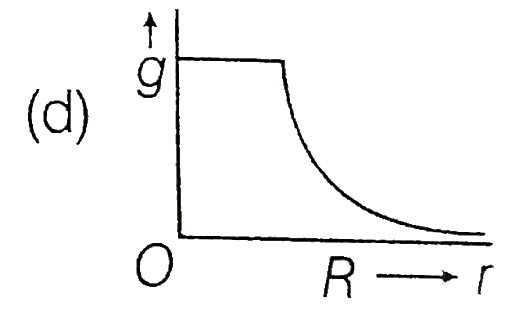572